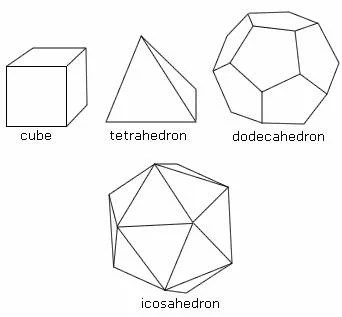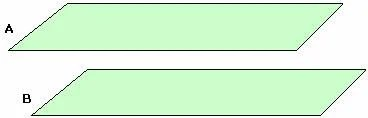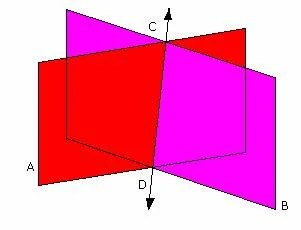## Prisms

Prisms are another common type of polyhedron. A prism has two parallel bases, upper and lower, which are congruent polygons. Like pyramids, prisms get their names from the shape of their bases. If lateral sides of a prism are perpendicular to the bases, as in the case of a triangular, quadrilateral, hexagonal, and rectangular prisms, they are rectangles. Such a prism is called a right prism. A rectangular prism, which modeled by a box, is the most common type of prism. If some of the lateral faces of a prism are parallelograms that are not rectangles, as in the pentagonal prism, the prism is called oblique prism. The union of a prism and its interior is called a solid prism. A rectangular prism that is a solid is sometimes called a rectangular solid.## Pyramids

When thinking of the word pyramid what generally comes to mind are structures built by the ancient Egyptians. Egyptian pyramids have a square base and triangular sides rising up to the vertex. This is just one type of pyramid. In general, the base of the pyramid can be any polygon, but its sides are always triangular. Pyramids are named according to the shape of their bases.## Regular Polyhedra

The best known of the polyhedra are the regular polyhedra, or platonic solids.  A regular polyhedron is a convex polyhedron whose faces are congruent regular polygons, the same number of which meet at each vertex. The ancient Greeks proved that there are only five regular polyhedra. The tetrahedron has 4 triangles for faces; the cube has 6 square faces; the octahedron has 8 triangular faces; the dodecahedron has 12 pentagons for faces; and the icosahedron has 20 triangular faces.The first four of the regular polyhedra can be found in nature as crystals of pyrite. The other regular polyhedron, the icosahedron, does not occur as crystals but has been found in the skeletons of microscopic sea animals called radiolarians.

## Polyhedra

The surface of a figure in space whose sides are polygonal regions, is called a polyhedron. The polygonal regions are called faces, and they intersect in the edges and vertices of the polyhedron. The union of a polyhedron and its interior is called a solid. A polyhedron is convex if the line segment connecting any two of its points is contained inside the polyhedron or on its surface.## Planes

In two dimensions, all figures occur in a plane. In three dimensions, there are an infinite number of planes. Each plane partitions space into three disjoint sets: the points on a plane and two half-spaces. Any two planes either are parallel, or intersect in a line.When two planes intersect, the angle between the planes is known as the dihedral angle.  A dihedral angle is measured by measuring the angle whose sides lie in the planes and are perpendicular to the line of intersection of the two planes.

## Relativity

The notion of space in geometry is an undefined term, just as the ideas of point, line, and plane are undefined. We intuitively think of space as three-dimensional and plane as only two-dimensional. In his history of relativity, Einstein tied together the three dimensions of space and the forth dimension of time. He showed that space and time affect each other and give us a four-dimensional universe.## Congruence

The idea of congruence is rather simple. Two plane figures are congruent if one can be placed on the other so that they coincide. Another way to say it is that they have the same shape and size.Two line segments are congruent if they have the same length, and two angles are congruent if they have the same measure.## Polygon and Tessellations

A polygon is a simple closed curve that is the union of line segments. Polygons are classified according to the number of line segments. The line segments of a polygon are called sides, and the endpoints of these segments are called vertices. Two sides of a polygon are adjacent sides if they share a common verex, and two verticies are adjacent vertices if they share a common side.In a regular polygon all of the sides are the same length and all of the angles have the same degree measurment. For example: a pentagon has five sides all the same length, and all of the interior angles are the same with a measurement of 108°.

There are three special angles in regular polygons. A vertex angle is formed by two adjacent sides of the polygon; a central angle is formed by connecting the center of the polygon to two adjacent vertices of the polgon; and an exterior angle is formed by one side of the polygon and the extension of an adjacent side.Any arrangement in which nonoverlapping figures are placed together to entirely cover a regionis called a tessellation. Floors and ceilings are often tessellated, or tiled, with square-shaped material, becuase squares can be joined together without gaps or overlaps. Equilateral triangles are also commonly used for tessellations. These three types of polygons– regular hexagons, squares, and equilateral triangles– are the only regular polygons that will tessellate.## Undefined Terms

A mathematical system consists of undefined terms, definitions, axioms, and theorems.  There must always be words that are undefined, words in this chapter that are undefined are point and line. We all have an idea of what a line is, but trying to define it proves to be difficult, and may invovle more words, such as straight, extends indefinitely, and has no thickness. The words used to describe the line also need to be defined. Point and line are used in definitions to describe other words.The definitions given in the book for point and line are: “Points are abstract ideas, which we illustrate by dots, corners of boxes, and tips of pointed objects. These concrete illustrations have width and thickness, but points have no dimension. A line is a set of points that we describe intuitivley as being “straight” and extending indefinitely in both directions.”